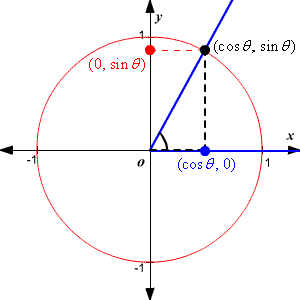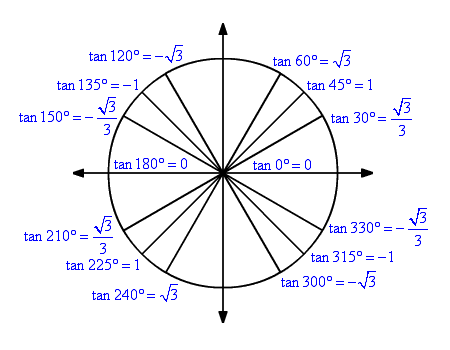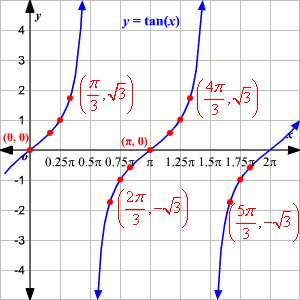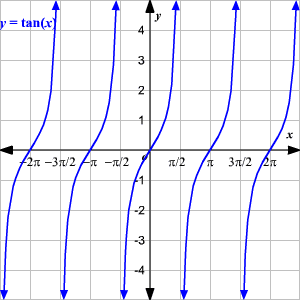# Tangent Function

The tangent function is a periodic function which is very important in trigonometry.

The simplest way to understand the tangent function is to use the unit circle. For a given angle measure $\theta$ draw a unit circle on the coordinate plane and draw the angle centered at the origin, with one side as the positive  $x$ -axis. The  $x$ -coordinate of the point where the other side of the angle intersects the circle is $\mathrm{cos}\left(\theta \right)$ and the  $y$ -coordinate is $\mathrm{sin}\left(\theta \right)$ .There are a few sine and cosine values that should be memorized, based on $30°-60°-90°$ triangles and $45°-45°-90°$ triangles. Based on these, you can work out the related values for tangent.

 $\mathrm{sin}\left(\theta \right)$ $\mathrm{cos}\left(\theta \right)$ $\mathrm{tan}\left(\theta \right)$ $\mathrm{sin}\left(0°\right)=0$ $\mathrm{cos}\left(0°\right)=1$ $\mathrm{tan}\left(0°\right)=\frac{0}{1}=0$ $\mathrm{sin}\left(30°\right)=\frac{1}{2}$ $\mathrm{cos}\left(30°\right)=\frac{\sqrt{3}}{2}$ $\mathrm{tan}\left(30°\right)=\frac{1}{2}\cdot \frac{2}{\sqrt{3}}=\frac{\sqrt{3}}{3}$ $\mathrm{sin}\left(45°\right)=\frac{\sqrt{2}}{2}$ $\mathrm{cos}\left(45°\right)=\frac{\sqrt{2}}{2}$ $\mathrm{tan}\left(45°\right)=\frac{\sqrt{2}}{2}\cdot \frac{2}{\sqrt{2}}=1$ $\mathrm{sin}\left(60°\right)=\frac{\sqrt{3}}{2}$ $\mathrm{cos}\left(60°\right)=\frac{1}{2}$ $\mathrm{tan}\left(60°\right)=\frac{\sqrt{3}}{2}\cdot \frac{2}{1}=\sqrt{3}$ $\mathrm{sin}\left(90°\right)=1$ $\mathrm{cos}\left(90°\right)=0$ $\mathrm{tan}\left(90°\right)=\frac{1}{0}=\text{undef}.$

Note that:

• for angles with their terminal arm in Quadrant II, since sine is positive and cosine is negative, tangent is negative.
• for angles with their terminal arm in Quadrant III, since sine is negative and cosine is negative, tangent is positive.
• for angles with their terminal arm in Quadrant IV, since sine is negative and cosine is positive, tangent is negative.You can plot these points on a coordinate plane to show part of the function, the part between $0$ and $2\pi$ .For values of $\theta$ less than $0$ or greater than $2\pi$ you can find the value of $\theta$ using the reference angle .

The graph of the function over a wider interval is shown below.Note that the domain of the function is the whole real line and the range is $-\infty \le y\le \infty$ .